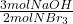## Consider the chemical equation. 2NBr3 + 3NaOH Right arrow. N2 + 3NaBr + 3HOBr If there are 40 mol of NBr3 and 48 mol of NaOH, what is the ex

Question

Consider the chemical equation. 2NBr3 + 3NaOH Right arrow. N2 + 3NaBr + 3HOBr If there are 40 mol of NBr3 and 48 mol of NaOH, what is the excess reactant?

in progress 0
1 month 2021-08-13T20:49:58+00:00 1 Answers 1 views 0

NBr₃ is the excess reactant.

Explanation:

• 2NBr₃ + 3NaOH → N₂ + 3NaBr + 3HOBr

We can solve this problem by calculating how many moles of NaOH would react completely with 40 moles of NBr₃, using the stoichiometric coefficients of the reaction:

• 40 mol NBr₃ *=60 moles NaOH

As the required number of NaOH moles is higher than the available number (60 required vs 48 available), NaOH is the limiting reactant.

As such, the excess reactant is NBr₃.